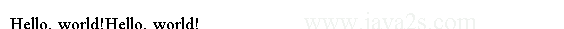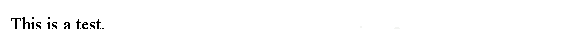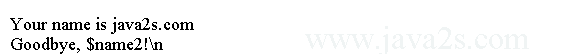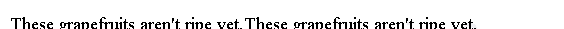# PHP Tutorial - PHP Introduction

PHP scripts are generally saved with the file extension `.php`.

## Statement

The basic unit of PHP code is called a statement, which ends with a semicolon.

Usually one line of code contains just one statement, but we can have as many statements on one line as you want.

## PHP Opening and Closing Code Islands

`<?php` and `?>` marks the PHP code island.

The short tags version is `<?` and `?>`.

`<?="Hello, world!" ?>`

Here is the equivalent, written using the standard open and closing tags:

```<?php
print "Hello, world!";
?>
```

## Example - PHP Statements

The following PHP code uses print statement to output message on to the screen.

```<?php
// option 1
print "Hello, ";
print "world!";

// option 2
print "Hello, "; print "world!";
?>
```

The code above generates the following result.`echo` is another command we can use to output message. `echo` is more useful because you can pass it several parameters, like this:

```<?php
echo "This ", "is ", "a ", "test.";
?>
```

The code above generates the following result.To do the same using print, you would need to use the concatenation operation (.) to join the strings together.

## PHP Variables

A variable is a container holding a certain value.

## Syntax

Variables in PHP beginning with `\$` followed by a letter or an underscore, then any combination of letters, numbers, and the underscore character.

Here are the rules we would follow to name variables.

• Variable names begin with a dollar sign ( `\$` )
• The first character after the dollar sign must be a letter or an underscore
• The remaining characters in the name may be letters, numbers, or underscores without a fixed limit

## Example - Define PHP variables

We cannot start a variable with a number. A list of valid and invalid variable names is shown in the following table.

Variable Description
\$myvar Correct
\$Name Correct
\$_Age Correct
\$___AGE___ Correct
\$Name91Correct;
\$1Name Incorrect; starts with a number
\$Name'sIncorrect; no symbols other than "_" are allowed

Variables are case-sensitive. `\$Foo` is not the same variable as `\$foo`.

## Variable substitution

In PHP we can write variable name into a long string and PHP knows how to replace the variable with its value. Here is a script showing assigning and outputting data.

```<?php
\$name = "java2s.com";
\$name2 = \$name;
print 'Goodbye, \$name2!\n';
?>
```

The code above generates the following result.PHP will not perform variable substitution inside single-quoted strings, and won't replace most escape characters.

In the following example, we can see that:

• In double-quoted strings, PHP will replace `\$name` with its value;
• In a single-quoted string, PHP will output the text `\$name` just like that.
```<?php
\$food = "grapefruit";
print "These \${food}s aren't ripe yet.";
print "These {\$food}s aren't ripe yet.";
?>

```

The code above generates the following result.The braces {} tells where the variable ends.